# Modeling The Capacity Factor of Analytes in Micellar Electrokinetic Chromatography Using Computational Descriptors

Document Type : Research Paper

Authors

1 Department of Medicinal Chemistry, School of Pharmacy, Tabriz University of Medical Sciences, Tabriz, Easthern Azarbayjan, Iran,

2 School of Pharmacy, Pharmaceutical Research Center, Tabriz University of Medical Sciences, Tabriz, Iran, 51664

3 Kimia Research Institute, Tabriz, Iran, P.O. Box 51665-171

Abstract

A multiple linear regression model is proposed to calculate capacity factor of  analytes using structural features computed using HyperChem software. The  chemical descriptors of analytes were computed using HyperChem software and  regressed against the experimental capacity factors of analytes collected from the  literature. The absolute average percentage deviation (AARD) and individual percentage  deviation (IPD) were calculated as accuracy criteria. The accuracy of the  proposed method was compared with that of a previously reported linear solvation  energy relationship (LSER). The proposed method was tested on ten experimental  data sets and mean ± standard deviation of AARDs were 48.5 ± 20.4 and 130.1 ± 79.7, respectively, for the proposed and LSER models in which the mean  difference was statistically significant (pthree subgroups, i.e. £ 45%, 45%-90%, and >90 %, shows the superiority of the proposed model over the LSER. A significant improvement in capacity factor modeling was achieved and the improvement factor is about 2.7. The descriptors could be easily computed and the calculations are straightforward. Therefore, the model is suggested to be employed in practice, however, the efforts should be continued until providing more accurate models.

Keywords

#### Full Text

1. Introduction

Electrophoresis is a sepration technique in which the analytes are separated based on their mobilities in a conductive medium, usually an aqueous buffer, in response to an applied electric field. Capillary electrophoresis (CE) is a relatively new technique in analytical sciences, which separates charged species using high voltage (i.e. up to 30 KV and even more). In this method, when an electric field is applied to a capillary tube, the sample’s ions migrate as a result of two types of movements, i.e. electrophoretic and electroosmotic  mobilities.  Electrophoretic mobility is the ion’s response to the applied electric field. Cations move toward the negetively  charged cathod, anions move toward the  positively charged  anode,  and  neutral species, which do not respond to the electric field; migrate with the electroosmothic flow. Consequently,  the charged analytes in CE could be separetd from each other and the uncharged  analytes  move  altogether  with electroosmotic flow.  By  adding  a  surface active agent above its  critical micelle con- centration to the running buffer, this limita- tion of CE could be overcome. The resulted method  is  called  micellar  electrokinetic chromatography (MEKC). Uncharged molecules in MEKC are separated according to their partitioning between the aqueous phase and  the  micellar  phase  (pesudostationary phase).  In  the  case  of  charged  solutes,  a combination  of  partitioning  between  the phases and electrophoretic mobility is  the mechanisms of their separations.

The capacity factor (k’) or retention factor (k) in MEKC is defined as the ratio of total  amount of the analyte in the micellar phase (nmic.) to the amount in the running buffer (nbuf.), and can be calculated by:where  Kmic.-buf.   is  the  micelle-buffer partition coefficient, Vmic. and Vbuf. are volumes  of miecellar and buffer phases, tr, t0 and tmic. are migration times of the analyte, electroosmotic flow  and  micelles,  respectively [(1]). The k’ is the main characteristics of an analyte in MEKC and in order to predict the possibility of successful separation of an analyte in a given analytical condition, its  numerical  value  should  be  estimated. Despite the experimental determination of k’ values, it could be calculated using quanitative    structure     property    relationships (QSPRs). The QSPRs are able to predict k’ values after training by a minimum number of experimental data points which is called training set, and a pure predictive method (a method without any curve-fitting parameter) is not available so far. It should be also noted that the QSPR models are able to predict k’ values at the analytical conditions which the training data sets have been collected and by changing the analytical conditions, the models should be re-trained using new data sets. Although a number of equations were presented for  modeling  of the electrophoretic mobility data in CE [(2-6]), the only avail- able model for calculating capacity factor of analytes in  MEKC  is  the linear solvation relationship (LSER) model, which is used in the  literature  ([7-10]).  In  addition  to  the lowess accuracy and relatively the high per- centage errors of LSER, the numerical values of its descriptors are not easily available for most of the pharmaceutical compounds. The aim of this work is to present a simple least squares model for calculating k’ of analytes using computational  descriptors. The accuracy of the proposed model is checked employing  experimental  k’  data  collected from the literature and, it is compared with that of a previously published model.

2. Experimental data and computational methods

2.1. Experimental data

The eExperimental data of capacity factors of pharmaceutically/chemically-interested compounds was collected from the literature. The details of the data were given in Tables 1 to -4. In Table 2, for a number of analytes, using different surfactants, the numerical values of k’ arewas reported equal to zero which means that the analyte moves with electroosmotic flow, and there is no tendency to the micelles. These data points were excluded from the calculations, since ln (0) is not a defined value.

Table 1. Details of analytes, experimental logk’ values (8) and the chemical descriptors.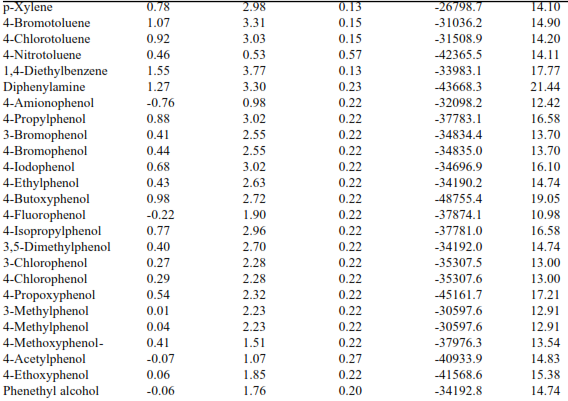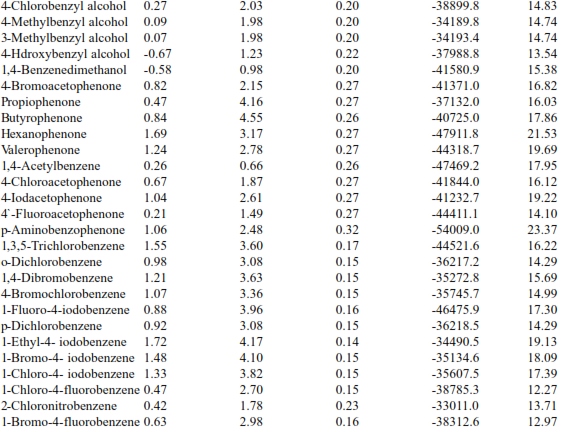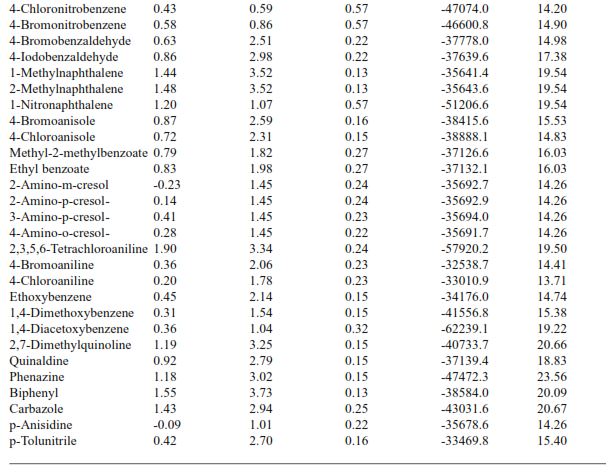a k’ values determined using 40 mM SDS in 20 mM NaH2PO4 at pH=7.0 or 12.0 [(8]).

Table 2. Details of analytes, experimental logk’  values (10) and the chemical descriptors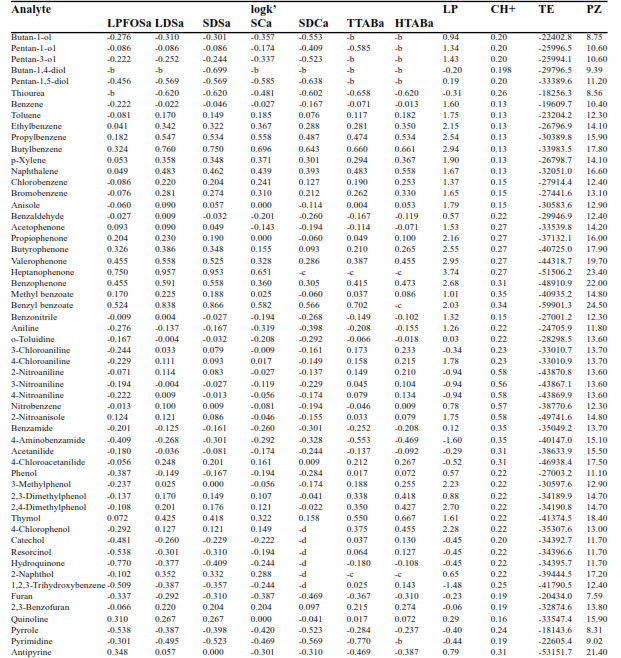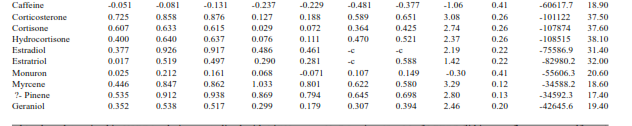a k’ values determined in H3PO4 solution neutralized with LiOH up to pH=7.0 using 40 mM of LPFOS (lithium perfluorooctanesulfonate) and 40 mM of LDS (lithium dodecyl sulfate), in sodium phosphate buffer at pH=7.0 using 40 mM of SDS (sodium dodecyl sulfate), 80 mM of SC (sodium cholate), 20 mM of TTAB (tetradecyltrimethylammonium bromide) and 20 mM of HTAB (hexadecyltrimethylammonium bromide) and in sodium phosphate-sodium tetraborate buffer at pH=8.0 using 40 mM of SDC (sodium deoxycholate). Dodecanophenone and methanol were used as micellar and electroosmotic flow markers and were excluded from the calculations.
b The roprted k’ values are ~0 (10) and were excluded from the calculations.
c The analytes coelute with dodecanophenone and their k’ values were not reported roprted in the reference (10).
d Analytes with pKa values between 9 and 10 and therefore, partially ionized at pH=8.0 (10).

2.2. Computational methods

2.2.1. Calculation of the descriptors

More detailed structural information of the studied compounds was calculated using molecular and quantum mechanical calculation methods. The theoretical descriptors (containing hydrophobic, electronic, theoretical and steric descriptors) for each compound were computed using AM1 semi-empirical quantum mechanical method by employing molecular descriptors, properties and orbital programs of HyperChem 7.0 [(11]). The structure of each compound was drawn in 2D and was converted to 3D using HyperChem 7.0 [(11]), and pre-minimized by Polak-Ribiere (12) geometry optimization using MM+ [(12). The structures were found by MM+, used as the starting point for re-minimization by Polak-Ribiere optimization using AM1 [(13]) semi-empirical quantum mechanical method. The calculated descriptors were octanol-water partition coeffi- cient, positive and negative partial charges on the analytes, accessible surface area, molar volume, hydration energy, molar refractivity, polarizability, dipole moment, total energy and heat of formation.

2.2.2. Statistical analysis

The calculated descriptors and the collected capacity factors were stored in SPSS data files and were analyzed using SPSS (version 10.0) software. To select the descriptors for including in the model, the Pearson correlation of log k’ and the descriptors were studied and the overall mean of correlation coefficients of 10 studied sets was considered along with the correlation matrix of the descriptors. The selection criteria were the highest mean of the correlation coefficients between log k’ and the descriptors and the lowest mean of inter corelations between the descriptors.

To provide a single multi-linear model, the selected descriptors were included in the model building process using ENTER subcommand of SPSS.

A number of diagnostic criteria are available to check the performance of a model. The most widely used criterion is the coefficient of determination (R2) or the correlation coefficient (R). It is calculated from the regression sum of squares and the total sum of squares. The significance of R2 and R values could be checked using F value and its associated probability. These criteria give useful information regarding the performance of the model. However, one could not compare the calculated k’ values with the experimental values. In addition, the numerical values of R and F could be affected by logarithmic or other mathematical transformations on the dependent variable, the number of inde- pendent variables included in the model and also the number of data points in each set. Therefore, to test the correlation ability of the proposed model, all data points in each set were fitted to the model and the back- calculated capacity factors were compared with the corresponding experimental values and the absolute average relative deviation (AARD) was computed as an accuracy criterion, by:

where N is the number of data points in each set. The main advantage of AARD is that, it is a comparable quanitity with the experimentally obtained relative standard deviation (RSD) of repeated experiments. To study the individual percentage deviations (IPD) of each data, the IPDs were computed using:

To  test the  stability of  the  correlation ability of the proposed model, the cross-validation   by   one-leave-out   method   was employed.  To  do  this,  one  datum  was excluded from the set and the remaining (N-1)  data  points  were  correlated.  Then  the back-calculated mobilities for each run of the  analysis  were  used  to  compute  the AARD term. Less variation in AARD values for the runs means that the model is robust and taking out one datum and/or addition of one more datum could not affect its accuracy.

2.2.3. The proposed model

The correlation of the calculated descriptors with the logarithm of the capacity factor (logk’) were studied considering above mentioned statistical criteria, and the following equation is proposed to provide better accuracy for modeling capacity factor of analytes in MEKC. The model is: wWhere J0-J4 are the model constants computed using a least squares analysis and their numerical values represent the interactions between the set of analytes and the

buffer and/or micelles, LP is the logarithm of partition coefficient, TE represents the total energy, PZ stands for polarizability and CH+ is the partial positive charge on the analyte. The previously presented LSER model [(7-10]) is:

w Where c, v, r, s, a, and b are the model.

The correlation of the calculated descriptors with the logarithm of the capacity factor constants, Vx is the McGowan’s characteris (logk’) were studied considering above mentioned statistical criteria, and the following equation is proposed to provide better accuracy for modeling capacity factor of analytes in MEKC. The model is:

wWhere J0-J4  are the model constants computed using a least squares analysis and their numerical values represent the interactions between the set of analytes and the tics volume, R2 is the excess molar refractivity, is the analyte’s dipolarity/polarizability, and  are the analyte’s hydrogen bond acidity and basicity, respectively. The basic mechanism of the analyte’s retention in MEKC is the partitioning between the buffer and the micelles. Both models represents the possible interactions in the solution using different descriptors of  the  analytes. The LSER model has been employed to represent other phenomena  such  as  retention  in  reversed phase liquid chromatography [(14]) and to the best of our knowledge, it is the only multiple linear regression model for explaining the capacity factor in MEKC. The calculated k’ values  for  the  studied  data  sets  using LSER model were used to compare with the corresponding calculated values  using  the proposed model.

3. Results and Discussion

All data points in each experimental data set were fitted to the equations IV and V, and the back-calculated k’ values were used to compute AARD values which arewere listed in Tables 5 and 6 along with the corresponding model constants. The proposed model shows the the lowest and the highest AARD values of 26.5% and 88.9 % were for the set numbers 2 and 9, respectively, whereas the corresponding values for the LSER model were 16.4% and 252.9 % for the set numbers 11 and 9, respectively. As it is evident from the results shown in Table 5, the proposed model provide better calculated k’ values. Figure 1 shows the overall mean and standard deviations of the AARD values for the studied data sets. The difference between the mean  of  AARDs  of  the  proposed  model (48.5 ± 20.4) with the LSER model (130.1 ±79.7) reveal that the difference is statistically significant (paired t-test, p Figure 2 shows the IPD distribution for the proposed and LSER models sorted in three    subgroups,    i.e.    IPD    ≤    45%, 45%90 %. The pro- posed model is able to compute k’ values with error < 45 in 69 % of the cases, where- as  the  correponding  value  for  the  LSER model is 42 %. The frequency of the IPD>90 % for the proposed and LSER models are  8  and 32  %,  respectively. Comparing these results reveal that the proposed model is able to provide better calculations in comparison with the LSER model. In addition, its descriptors could be easily computed by HyperChem using the chemical structure of the analytes of interest. In comparing the proposed model with the LSER, one should keep in mind that the LSER possesses six curve-fitting parameters, whereas those of the proposed model is five, and this could be considered as another advantage of the pro- posed model.

Figure 1. Mean and standard deviation of AARD values for the proposed and LSER models.

Figure 2. Distribution of individual percent deviation of the models studied.

Table 3. Details of analytes, experimental logk’ values [(9]) and the chemical descriptors.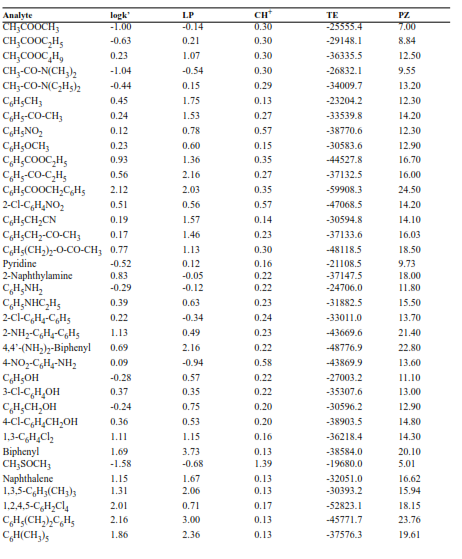Table 4. Details of analytes, experimental logk’ values [(7]) and the chemical descriptors.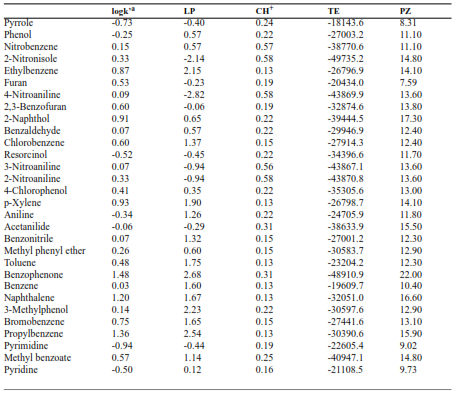ak’ values determined in sodium phosphate-sodium tetraborate, pH=8.0 using 50 mM of SDS (sodium dodecyl sulfate).

Table 5. Absolute average relative deviation (AARD), the model constants and their standard errors (s.e.) of the proposed model for studied sets.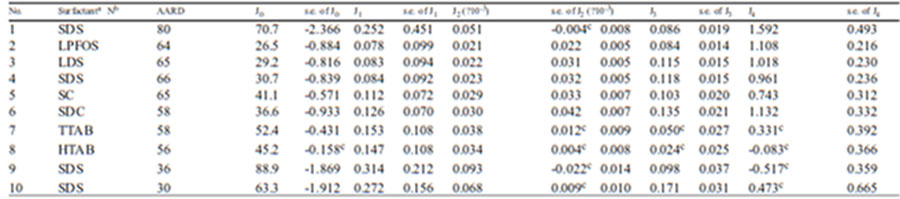Table 6. Absolute average relative deviation (AARD) and the model constants of the LSER model for studied sets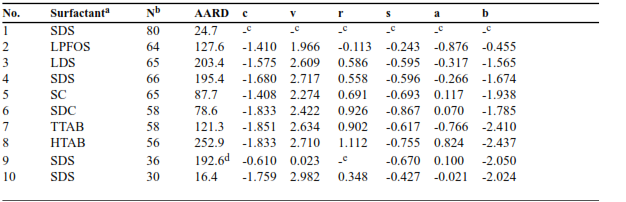a  Other experimental details are the same as reported in Tables 1-4.

b N is the number of data in each set.

c The model constants were not given in the reference [(8]) and the APD values were computed using predicted reported k’

values [(8]).

d The APD values calculated using the reported LSER constants in the reference gives APD of 5720.8 %, and it should be

due to a typographical error in reporting the model constants. We used the reported LSER constants with opposite signs and obtained 192.6

Once the model is trained using a limited number of experimental data points, the trained model could be used to predict the unmeasured k’ values and the possibility of successful separation using the MEKC sys- tem under investigation could be predicted forcasted.  The  main  limitation  of  this method (and also LSER model) is that the models require a  number of  experimental data  for  training  process,  and  the  trained models are only valid for the same analytical conditions which training points are collect- ed.  and  Aanother model should be trained when one or more variables of MEKC sys- tem  arewere modified. To evaluate the stability of the proposed model, one- leave out method  was  employed  and  the  obtained results showed that there are no significant changes in the numerical values of the model constants and also AARD values, and these mean  that the model is robust and adding and/or deleting one datum in a data set could not affect the accuracy of the model. The produced AARD by the proposed model is relatively high, when it is compared to the experimental  relative  standard  deviation (RSD) for repeated experiments. However, it is a fact that the proposed model is able to improve the accucary of k’ calculations by a factor of 2.7 and is a step forward in MEKC data modeling. It is obvious that the efforts should be continued until providing a more accurate  model  with  AARD  comparable with RSD values.

#### References

   Herbert BJ, Dorsey JG., n-Octanol-water partition coefficient estimation by micellar electrokinetic  capillary  chromatography., Anal. Chem.1995; 67: 744-749.
   McKillop AG, Smith RM, Rowe RC, Wren AC., Modeling  and  prediction  of  electrophoretic mobilities     in     capillary     electrophoresis: Separation of alkylpyridines., Anal. Chem. 1999;71: 497-503.
   Jalali-Heravi M, Garkani-Nejad Z., Prediction of electrophoretic   mobilities    of    alkyl-    and alkenylpyridines  in  capillary  electrophoresis using artificial neural networks., J. Chromatogr. A 2002; 971: 207-215.
   Liang HR, Vuorela H, Vuorela P, Riekkola MJ, Hiltunen R., Prediction of migration behaviour of  flavonoids in capillary zone electrophoresis by means of topological indices., J. Chromatogr. A 1998; 798: 233-242.
   Li Q, Dong D, Jia R, Chen X, Hu Z, Fan BT.,Development of a quantitative structure-proper-
ty relationship model for  predicting the elec- trophoretic mobilities., Comp. Chem. 2002; 26:
245-251.
   Jouyban A, Yousefi BH., A quantitative structure property  relationship  study  of  electrophoretic mobility   of  analytes  in  capillary  zone  elec- trophoresis.,  Comput.  Biol.  Chem.  2003;  27:297-303.
   Roses  M,  Rafols C,  Bosch  E,  Martinez AM, Abraham  MH.,  Solute-solvent  interactions  in micellar       electrokinetic      chromatography. Characterization of sodium dodecyl sulfate-Brij
35 micellar systems for quantitative structure- activity relationship modeling. J. Chromatogr. A
1999; 845: 217-226.
   Kelly KA, Burns ST, Khaledi MG., Prediction of retention in micellar electrokinetic chromatogra- phy  from solute structure. 1. Sodium dodecyl sulfate micelles. Anal. Chem. 2001; 73: 6057-6062.
   Taillardat-Bertschinger A, Carrupt PA, Testa B., The relative partitioning of neutral and ionized compounds in sodium dodecyl sulfate micelles measured  by  micellar  electrokinetic  capillary chromatography.  Eur. J. Pharm. Sci. 2002; 15:225-234.
 Fuguet E, Rafols C, Bosch E,, Abraham MH, Roses M., Solute-solvent interactions in micellar electrokinetic          chromatography.          III. Characterization of the selectivotyy of micellar electrokinetic   chromatography   systems.   J. Chromatogr. A 2002; 942: 237-248.
 HyperChem  7.0.,  Molecular  mechanics  and quantum chemical calculations package, 2002,